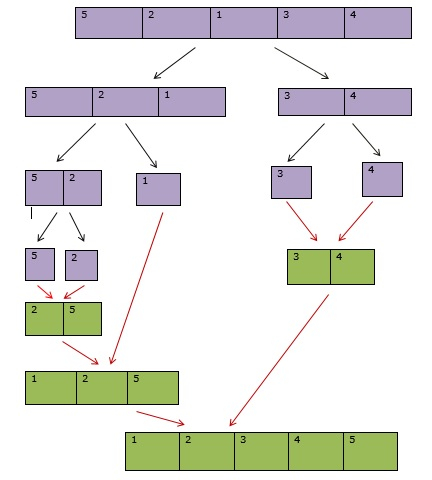# Explain Merge Sort in Python

Merge sort is a sorting technique. It is an efficient sorting algorithm with a time complexity of (n logn) where n is the length of the array to be sorted.

Merge sort is an algorithm that follows the Divide and Conquers paradigm. It continuously divides the array into two equal halves. Later it starts sorting the lists having a single element each and continuously merges the sorted lists to form the complete sorted list.

Hence, we obtain a sorted array.

ExampleThe purple boxes and black arrows show the splitting of the list into two halves.

The green boxes and red arrows show the merging of the two sorted lists.

## Implement Merge Sort

Splitting the list into two halves is quite easy and it is done recursively until we have only one element left. Later the merging procedure is done which is actually where we apply the logic of merging the two sorted lists together.

### Example

The merge function takes the two sorted arrays to be merged. The frontmost element of a1 is compared with the frontmost element of a2. The smallest of two is added to list c and the pointer of that array is incremented.

Live Demo

def merge(a1,a2):
c=[]
x=0
y=0
while(x<len(a1) and y<len(a2)):
if(a1[x]<a2[y]):
c.append(a1[x])
x+=1
else:
c.append(a2[y])
y+=1
while(x<len(a1)):
c.append(a1[x])
x+=1
while(y<len(a2)):
c.append(a2[y])
y+=1
return c

def mergesort(array):
if(len(array)==1):
return array
mid=(len(array))//2
a1=mergesort(array[:mid])
a2=mergesort(array[mid:])
return merge(a1,a2)
array=[2,3,1,5,4,6,8,10,7,9]
print(mergesort(array))

### Output

[1, 2, 3, 4, 5, 6, 7, 8, 9, 10]